# 基于SimStore的核电站热力系统仿真Simulation of Thermal System of Nuclear Power Plant Based on SimStore

DOI: 10.12677/NST.2019.73015, PDF, HTML, XML, 下载: 449  浏览: 1,464  国家科技经费支持

Abstract: Based on the SimStore software package, the conventional island thermal system of the nuclear power plant of Bay Daya in Guangdong Province is taken as the simulation object in this paper. According to the mass conservation and energy conservation under the full load state, the matrix method and constant power output method are used to build a simulation model for typical load steady-state simulation and 70% - 100% load rise simulation. When the simulation system is running stably under 100%, 90% and 70% loads, the accuracy is acceptable; in the load lifting simulation of 70% - 100%, the flow and pressure changes of the main equipment in the system are logical. Reference is provided for the operation, simulation, optimization, the improvement of the efficiency of relevant education and practical training of the conventional island thermal system of nuclear power plant and the development of offshore nuclear power platform.

1. 引言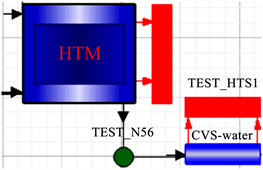Figure 1. Local diagram of simulation model

2. 仿真模型热力参数计算方法

2.1. 满负荷下的热力参数计算方法

2.1.1. 效率计算法

2.1.2. 热平衡法及矩阵法

$N=\left({h}_{0}-{h}_{n}\right)-{\sum }_{i=1}^{m}{\alpha }_{i}\left({h}_{e,i}-{h}_{n}\right)$ (1)

${h}_{i}^{d}={h}_{e,i}-{h}_{n}$ (2)

$N=\left({h}_{0}-{h}_{n}\right)-{\left[{\alpha }_{i}\right]}^{\text{T}}{\left[{h}_{i}^{d}\right]}^{\text{T}}$ (3)

$\left[{\alpha }_{i}\right]={\left[{\alpha }_{1},\cdots ,{\alpha }_{i},\cdots ,{\alpha }_{m}\right]}^{\text{T}}$ (4)

$\left[{h}_{i}^{d}\right]={\left[{h}_{1}-{h}_{n},\cdots ,{h}_{i}-{h}_{n},\cdots ,{h}_{m}-{h}_{n}\right]}^{\text{T}}$ (5)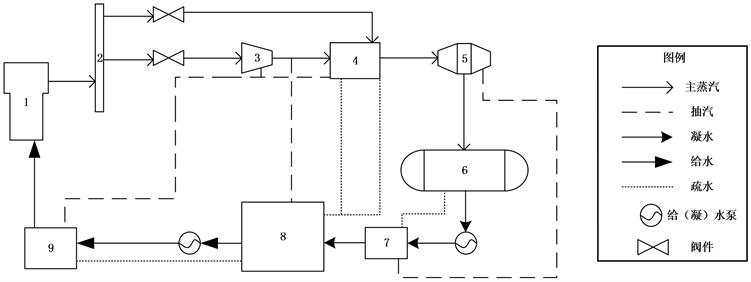1——蒸汽发生器；2——蒸汽母管；3、5——高、低压缸；4——汽水分离再热器；6——凝汽器；7、8、9、10——1#、2#、3#、4#低压给水加热器；11——除氧器；12#、13#——高压给水加热器。

Figure 2. Schematic diagram of thermal system

${\alpha }_{1}{q}_{1}={\tau }_{1}$ (6)

${\alpha }_{1}{\gamma }_{2}+{\alpha }_{2}{q}_{2}={\tau }_{2}$ (7)

$\left({\alpha }_{1}+{\alpha }_{2}\right){\gamma }_{3}+{\alpha }_{3}{q}_{3}={\tau }_{3}$ (8)

${\alpha }_{4}{q}_{4}=\left(1-{\alpha }_{3}-{\alpha }_{2}-{\alpha }_{1}\right){\tau }_{4}$ (9)

${\alpha }_{4}{\gamma }_{5}+{\alpha }_{5}{q}_{5}=\left(1-{\alpha }_{3}-{\alpha }_{2}-{\alpha }_{1}\right){\tau }_{5}$ (10)

${\alpha }_{6}{q}_{6}=\left(1-{\alpha }_{5}-{\alpha }_{4}-{\alpha }_{3}-{\alpha }_{2}-{\alpha }_{1}\right){\tau }_{6}$ (11)

${\alpha }_{7}{q}_{7}=\left(1-{\alpha }_{5}-{\alpha }_{4}-{\alpha }_{3}-{\alpha }_{2}-{\alpha }_{1}\right){\tau }_{7}$ (12)

$A\left[{\alpha }_{i}\right]=\left[{b}_{i}\right]$ (13)

2.2. 非满负荷下的热力参数计算方法

$\frac{{G}_{2}}{{G}_{1}}=\sqrt{\frac{{p}_{{2}_{1}}^{2}-{p}_{{2}_{2}}^{2}}{{p}_{{1}_{1}}^{2}-{p}_{{1}_{2}}^{2}}\frac{{T}_{{0}_{2}}}{{T}_{{0}_{1}}}}$ (14)

$f{\left(p,G,T,x\right)}_{i}=\frac{{G}_{2}{}_{i}}{{G}_{1}{}_{i}}\sqrt{\frac{{\left({p}_{{2}_{1}}+\frac{{a}_{{p}_{{2}_{1}}}}{{v}_{{m}_{2}}{}_{1}^{2}}\right)}^{2}-{\left({p}_{{2}_{2}}+\frac{{a}_{2}}{{v}_{{m}_{2}}{}_{2}^{2}}\right)}^{2}}{{\left({p}_{{1}_{1}}+\frac{{a}_{{p}_{{1}_{1}}}}{{v}_{{m}_{1}}{}_{1}^{2}}\right)}^{2}-{\left({p}_{{1}_{2}}+\frac{{a}_{{p}_{{1}_{2}}}}{{v}_{{m}_{1}}{}_{2}^{2}}\right)}^{2}}\frac{{T}_{{0}_{2}}}{{T}_{{0}_{1}}}}$ (15)

${f}_{c}=|\frac{f{\left(p,G,T,x\right)}_{a}}{f{\left(p,G,T,x\right)}_{b}}-1|$ (16)

3. 仿真结果与分析

3.1. 典型负荷稳态仿真3.2. 升负荷动态仿真

3.2.1. 主要设备流量的变化规律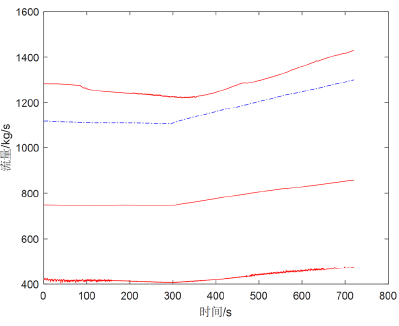Figure 3. Flow variation

3.2.2. 主要设备压力的变化规律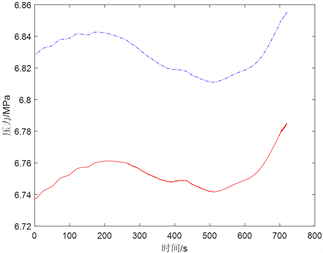(a)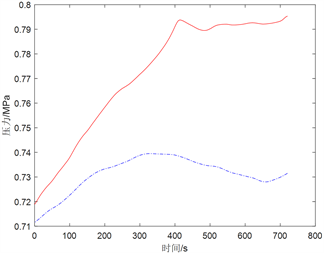(b)

Figure 4. Pressure variation; (a) The new steam pressure and the admission pressure of the high-pressure cylinder, (b) The inlet pressure of the low-pressure cylinder and the outlet feed pressure of the deaerator

4. 结语

  许世杰, 林萌, 杨燕华, 等. 基于核电站完整二回路的数学模型[J]. 核动力工程, 2010, 31(2): 50-53.  严思伟. 基于RELAP5的核电机组常规岛热力系统建模[D]: [硕士学位论文]. 南京: 东南大学, 2016.  张帆. 基于RELAP5的压水堆二回路全范围模拟及分析研究[D]. 北京: 华北电力大学, 2016.  郭亮, 孙宝芝, 宋志宇, 等. 基于C++Builder的核电站二回路热力系统建模与实时仿真[J]. 电力建设, 2013, 34(6): 1-6.  叶涛, 张燕平. 热力发电厂[M]. 北京: 中国电力出版社, 2017.  彭敏俊, 田兆斐. 核动力装置热力分析[M]. 哈尔滨: 哈尔滨工程大学出版社, 2012.  费景洲, 刘友, 王金鑫. 测试技术与虚拟仿真实验教程[M]. 哈尔滨: 哈尔滨工程大学出版社, 2017.  张小勇, 刘琦, 杨志平, 等. AP1000核电厂二回路热力系统计算与分析[J]. 热能动力工程, 2018, 33(9): 107-113.  苏森林, 杨辉玉. 900MW压水堆核电站系统与设备[M]. 北京: 原子能出版社, 2005.  聂万远, 方伟明. 船用核动力装置全系统特性仿真分析研究[J]. 舰船科学技术, 2018, 40(1): 94-98.  李勇, 杨磊磊. 汽轮机变工况各级热力参数计算方法研究[J]. 汽轮机技术, 2015, 57(5): 321-325+340.  祁琳, 赵守智, 王晓坤, 等. 中国示范快堆常规岛图形建模与仿真分析[J]. 原子能科学技术, 2017, 51(11): 1982-1988.  El-Sefy, M., Ezzeldin, M., El-Dakhakhni, W., Wiebe, L. and Nagasaki, S. (2019) System Dynamics Simulation of the Thermal Dynamic Processes in Nuclear Power Plants. Nuclear Engineering and Technology. https://doi.org/10.1016/j.net.2019.04.017  Jewer, S., Buchan, A.G., Pain, C.C. and Cacuci, D.G. (2014) An Immersed Body Method for Coupled Neutron Transport and Thermal Hydraulic Simulations of PWR Assemblies. Annals of Nuclear Energy, 68, 124-135. https://doi.org/10.1016/j.anucene.2013.12.018  Wang, Y., Li, Y., Cao, Y. and Zhang, T. (2018) Thermal-Fluid Coupling Simulation of Ventilation Cooling System of Generator Based on FLOWMASTER. Transactions of Nanjing University of Aeronautics and Astronautics, 35, 516-521.